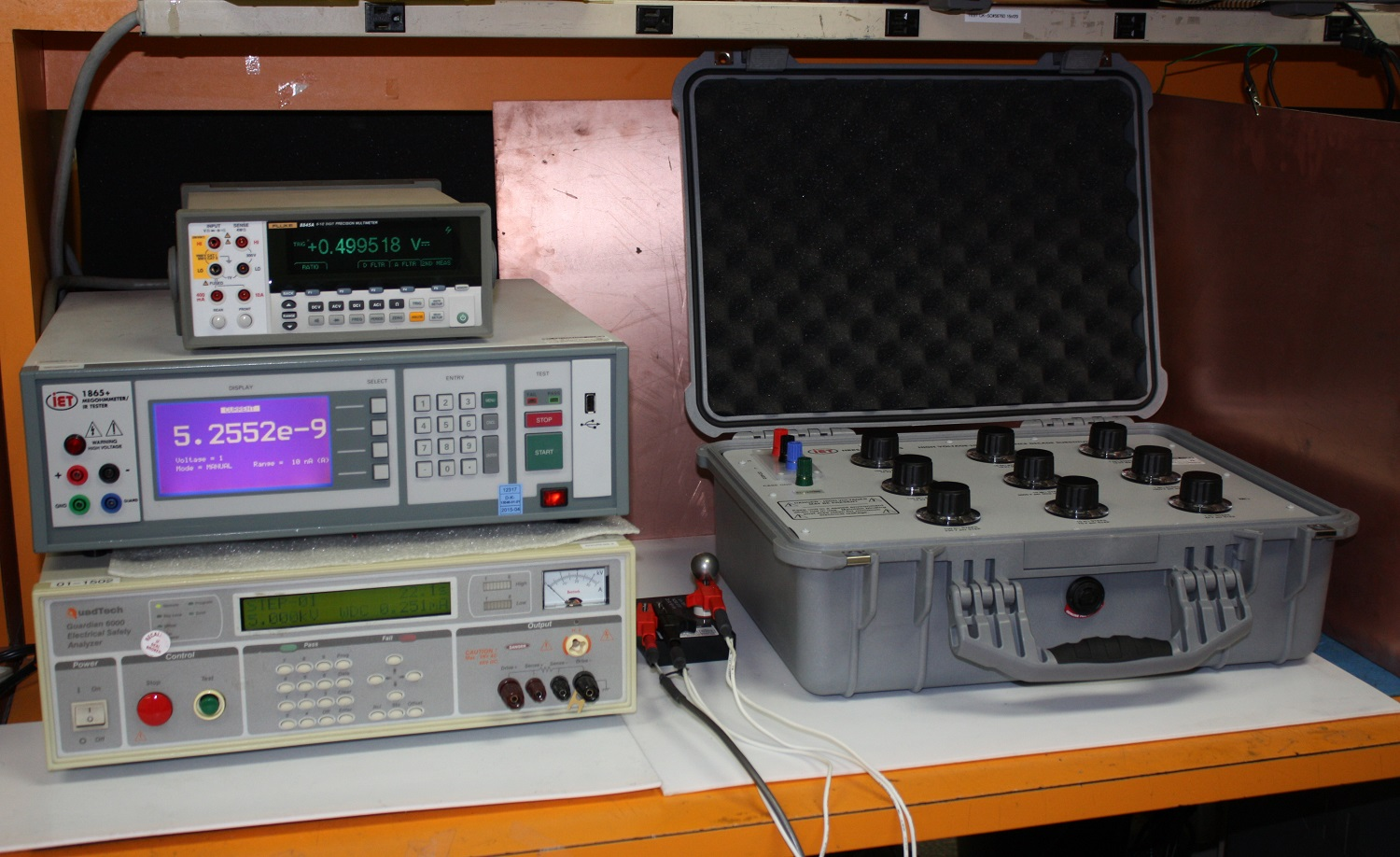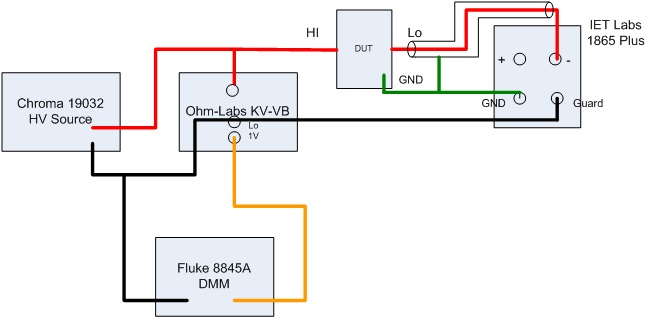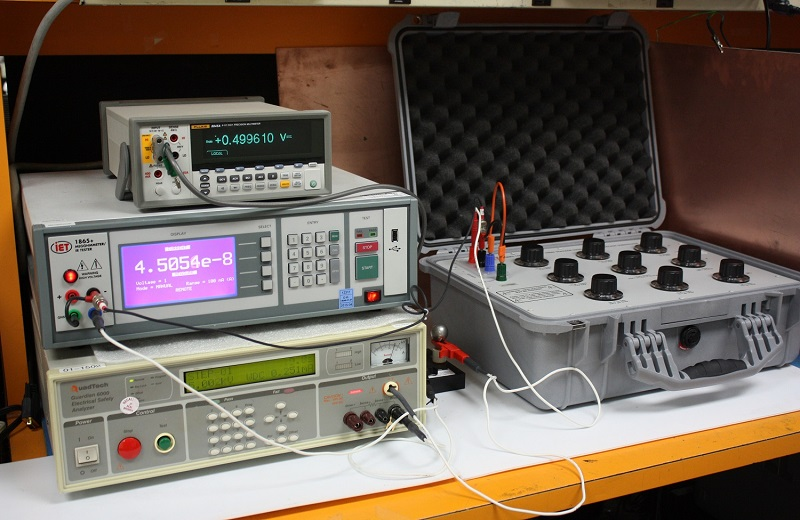# A system to measure two terminal high resistance standards at voltages up to 6 kVdc using the 1865 Plus Megohmmeter

The 1865 + Megohmmeter when combined with a high voltage dc source, DMM and voltage divider can create a system for measuring resistance at voltage up to 6 kVdc.

The concept was to use: a high voltage source that could produce up to 6 kVdc, measure the voltage across the device under test DUT and current flowing through the DUT. The resistance can then be calculated using Ohm’s Law. The system would be completely automated via a LabView program to configure the instruments, perform measurements, process the results and display the data.

The voltage and current would be sampled multiple times during the measurement time and resistance plotted over time along with the standard deviation of the average.

The system consists of the following instruments:
1. Chroma/QuadTech Guardian 6000 Electrical Safety Tester or current Chroma model 19032
2. Fluke 8845A 6 ½ Digit DMM
3. Ohm-Labs KV-VB Voltage Divider 10,000 to 1 (kvvb-compact-divider)
4. IET Labs 1865 Plus Megohmmeter 1865 + Megohmmeter
5. National Instruments LabView and GPIB-USB-HS adapter and a PC.Figure 1. High Voltage Resistance Measurement System

The 1865 Plus can be configured to measure and display current rather than just resistance. When measuring current the “-“ and Guard terminals are used. This is described further in the application note at the following link Using the 1865 Plus as a current meter .

Diagram 1. shows the interconnection of all pieces of the high voltage resistance measurement system. The instruments were chosen based upon accuracy, availability and capability of being controlled via a GPIB interface.Diagram 1. Interconnection Diagram of the High Voltage Resistance Measurement System

Figure 2. shows the high voltage resistance measurement system performing measurements at 5 kVdc. The DMM is measuring the voltage from 10000 to 1 voltage divider, so multiply the voltage shown by 10000 to determine the actual test voltage. The 1865+ shows the current flowing through the resistor under test. The resistance can then be calculated by voltage / current.Figure 2. High Voltage Resistance Measurement System Performing a Measurement

Figure 3. below shows the resistance graph that is displayed during the measurement. As the resistance value increases the overall test time as to be increased as well.

The graph aids in determining when the resistance measurement has stabilized. The display also shows the average of the resistance value and the standard deviation of the resistance over the final 25% of the measurement time.Figure 3. Graph of Resisistance vs Time with Average Resistance and Standard Deviation

This solution is capable of measuring high resistance standards from 10 MΩ to 1 TΩ at voltages above 1000 Vdc, with a measurement accuracy of < 0.5%.

The system can also measure resistance values up to 10 TΩ with a measurement accuracy of < 1%.

More detail on the system can be found at this Application Note. A system to measure high resistance at voltages up to 6 kVdc